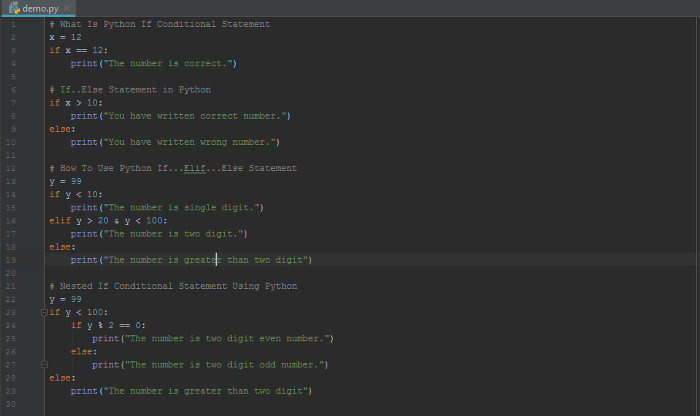# Python If Conditional Statement, If…else…If, Nested If

If you want to use the test conditions in Python, you have to use the Python if conditional statement. There are many types of if statement which you can learn here with the examples.

## What Is Python If Conditional Statement

It is the decision making the statement in Python programming works on the basis of conditions. You have to put the code inside the if statement. Also, put a valid condition in the Python if condition statement.

The entered code in the statement will only get executed if the condition is true. If the condition is not true, the code will not get executes. If you want to execute the single code with the single conditional statement. You have to use the single if condition when you have the only single condition to test.

You can also use the else statement with the code inside to execute when the condition is false. If the condition is false, the code inside the else statement will only get executed.Also, if you have more than one condition to test, you have to use multiple python If Conditional Statement using `ELIF` keyword. It may contain many codes inside each statement. However, only the single code will get executes which is inside the true if condition.

The syntax of If Condition

The above syntax shows that a simple if the condition requires only a single condition. It tests the condition and finds if it is true to execute the inner mentioned code.

However, if the condition is false, there are no other codes to execute. For this purpose, you have to use the if else condition which is the next section.

Example of if Condition

Output

The number is correct.

## If..Else Statement in Python

The if..else statement contains codes for both test result if true or false. If the condition is true, you will get the execution of the code inside the if statement. However, if the condition is not true, it executes the code under the else statement.

The syntax of if…else Condition

The above syntax contains the two codes inside the python If Conditional Statement and else statement. However, only the if statement contains the condition to test if true or false. If the condition is false, code under else statement will get executed.

See the example below to get the ideas of how to use the if…else condition.

Example of if…else Condition

Output

You have written correct number.

## How To Use Python If…Elif…Else Statement

If you want to use more than one test condition in the code. You have to use the if..elif..else condition statement. Under the elif keyword, you can also put a test condition to find if it is true or false. In addition to this, you can add else statement to execute the code if multiple test condition is false.

The syntax of if…elif…else Condition

The above syntax contains the two test condition to find if true of false. If any of the test condition is true, the code under the if condition of related test gets executed. You can add more than one elif keyword to add more test conditions in the above syntax.

The below example finds the two test condition if true or false.

Example of if…elif…else Condition

Output

The number is two digit.

The above example executes the code under the elif keyword. Because only the test condition in the elif statement is true.

## Nested If Conditional Statement Using Python

The nested if conditional statement contains the if condition inside another if condition. It mean, you can add if inside the if statement using Python. It contains the nested if with the code inside the innermost if condition.

If the first test condition is true, it test another if condition given inside. However, if the inner test if condition is not true, it executes the code under the else statement.

By using this condition, you can perform multiple test to check if true. It executes the code only if the mutliple inner if condition is true.

Syntax of Nested if Condition

The above syntax contains the if..else condition inside the if statement. You can also add the elif keyword to check the test case using the above syntax.

You can check the below given example to use the nested if condition in Python.
Example of Nested if Condition

Output

The number is two digit odd number.

After it checks and finds the outer and inner condition, it print the output as given above.

You may also like to read

Hope, you like this post of how to use the Python if condition and other statements. If you have any query regarding the tutorial, please comment below.

Also tell me, which method you are using for if statements in Python.## THE ULTIMATE TOOLKIT

Get FREE Access to Toolkit and Resources that we are using in Tutorialdeep that Every Professional Should Have! in one handy PDF.